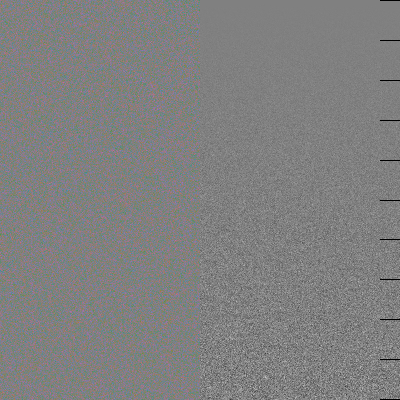# Chrominance vs Luminance noise comparison

Each of the images below is neutral gray modified by noise. The noise in the left half has constant chrominance component (different in different images, 4, 10, 20 and 40 in R'G'B' units), and practically no luminance component (one present is due to discretization errors; I expect it to be of order of magnitude 0.4). The right half has only luminance noise; on the top line it is 0, on the bottom line it is the same as the magnitude of noise in the LHS; the magnitude changes linearly with the height. Each tick on the right corresponds to 10% of the magnitude on the left.

Find the level on the right where visual noise to the eye "is comparable in strength" with the noise on the left. This shows the relative importance of chrominance noise w.r.t. the luminance noise for your eye. For myself, I do not think that the number is much above 10%.

More precise, the noise in the left half is normally distributed in the plane `LUMA=const`; if you take orthogonal coordinate system in this plane, two components of the noise are independent and normally distributed with the given number as the deviation. The noise in the right half is normally distributed on the line `R'=G'=B'`; the deviation of each coordinate is the given number.

Do not even think of trying to do this if your graphic system is below 24-bit. I do not expect that this may survive printing with less than perfect printers... I won't trust the data for noise=40 very much, since due to non-linearity of gamma-curves, high visible noise on the left may be due to difference of luma vs. luminosity; while luma is constant, luminosity has a significant noise there. This non-linearity may also explain visible red tint on the left hand side of the last graph.

`Noise=4``Noise=10``Noise=20``Noise=40`## HOWTO build this

The files were generated by the script random-noise.pl by running
``````
random-noise.pl 4  >rand4.png
random-noise.pl 10 >rand10.png
random-noise.pl 20 >rand20.png
random-noise.pl 40 >rand40.png
``````

Details of implementation: one call to `rand()` corresponds to one point on the left, and two points on the right. This system's `rand()` is BSD `random()`; it has 128 bits of internal state, and `randbits=31`.

Ilya Zakharevich; HOME